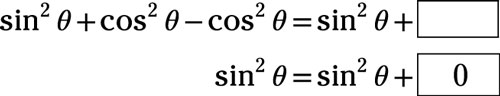##### Pre-Calculus: 1001 Practice Problems For Dummies (+ Free Online Practice)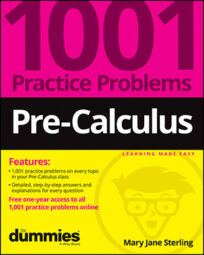The basic trig identities will get you through most problems and applications involving trigonometry. But if you’re going to broaden your horizons and study more and more mathematics, you’ll find some additional identities crucial to your success. Also, in some of the sciences, especially physics, these specialized identities come up in the most unlikely (and likely) places.

These trigonometric identities are broken into groups, depending on whether you’re trying to combine angles or split them apart, increase exponents or reduce them, and so on. The groupings can help you to decide which identity to use in which situation. Keep a list of these identities handy, because you’ll want to refer to them as you work through the problems.

You’ll work with the more-advanced trig identities in the following ways:

• Using the function values of two angles to determine the function value of the sum of the angles

• Applying the identities for the difference between two angles

• Making use of the half-angle identities

• Working from product-to-sum and sum-to-product identities

• Using the periods of functions in identities

• Applying power-reducing identities

• Deciding which identity to use first

When you’re working on these particular trig identities, some challenges will include the following:

• Applying the identities using the correct order of operations

• Simplifying the radicals correctly in half-angle identities

• Making the correct choices between positive and negative identities

## Practice problems

1. Use a sum or difference identity to determine the missing term in the identity: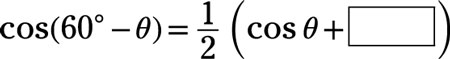Use the cosine-of-a-difference identity: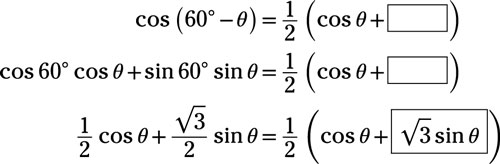2. Use a double-angle identity to determine the missing term in the identity.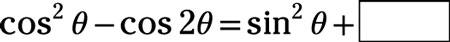Replace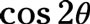with the double-angle identity involving the cosine: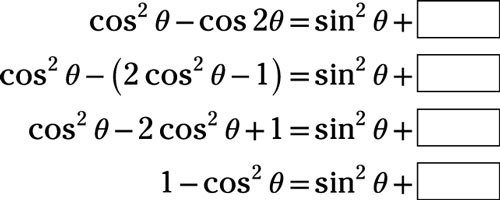Replace the 1 with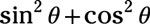from the Pythagorean identity: